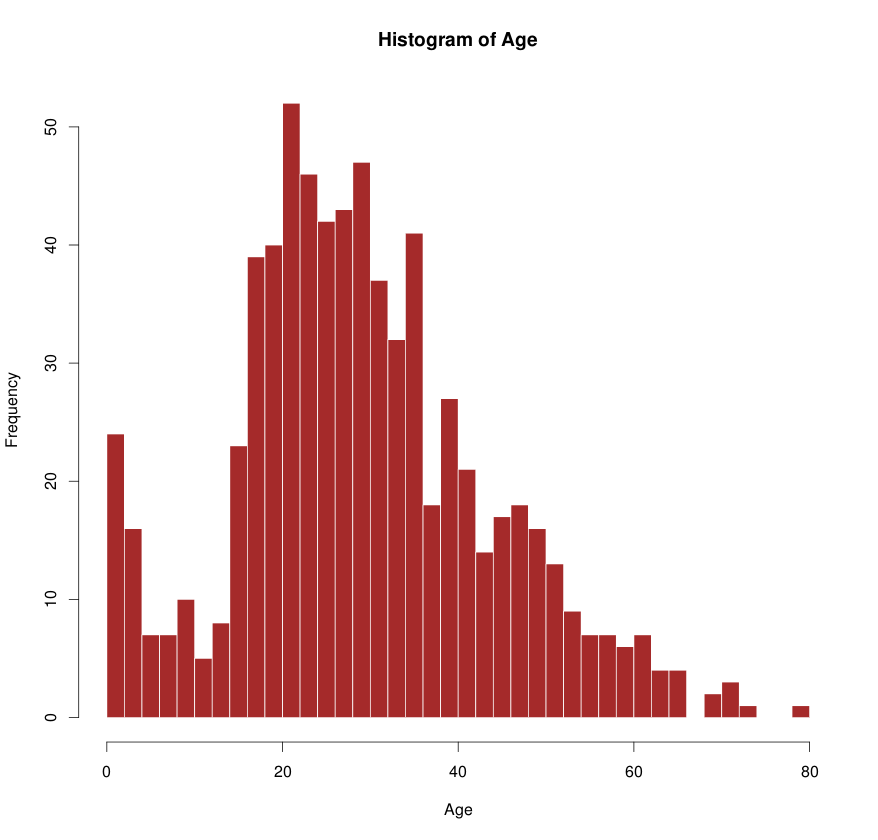SHORTS
☘☘☘ Letters by Vivek Rai

## Quick Data Plotting in R

Useful tips for exploratory data analysis

Python is my all time favorite programming language. I use it all the time. It is simple, readable and easy to get started, something which I picked up four years ago when I went through the first offering of Interactive Programming with Python course on Coursera. Python’s simplicity and appeal has continued to grow over time, supported by a wide community and a rich environment of packages.

Today, however, I am not going to talk about Python. This post shares some of my experiences with R programming language and how it helped me postpone my struggle with visualizations in Python (a short back-story at the end).

Plotting figures is real quick. An example below using the famous Titanic dataset from Kaggle competition.

``````data <- read.table(‘data.txt’, header=TRUE) # TSV file
names(d) # fetch columns
  "PassengerId" "Survived"   "Pclass"    "Name"        "Sex"
  "Age"         "SibSp"      "Parch"     "Ticket"      "Fare"
 "Cabin"       "Embarked"
``````

Looking at the columns, it would be interesting to explore the age distribution of the people, along with their survivor, class and sex distribution. Let’s take a look.

``````boxplot(Age ~ as.factor(Pclass), data=data)
title(xlab='Class', ylab='Age', main='Passenger age vs Class')
abline(h=median(data_Age, na.rm = TRUE), col='red')
``````

Boxplot is a good way to visualize the age distribution across passenger classes.

The histogram below is generated in a single line.While it may take a bit of time to find out which functions to use (most of the times they have intuitive names), it becomes super easy to generate complex figures by simply composing simple ones.

Also, when handling a large amount of data, plotting with a few tweaked parameters can save quite a bit of processing time. A few of these suggestions and helpful functions are compiled below with relevant examples.

## Functions

• `ifelse()`
``````col <- ifelse(dataSurvived == 1, 'red', 'gray'))
``````
• `boxplot()`
``````boxplot(Age ~ as.factor(Pclass), data=data)
``````
• `split()` - split dataframe, useful with `boxplot`
``````df <- split(data-Age, f='Pclass')
``````
• `hsv()` - generate colors
``````cols <- hsv((2/3)*as.integer(data-Pclass)/25, 1, 7/8)
``````
• `points()` - draw points on a graph
``````points(data\$Age, col=ifelse(dataSibSp > 0, 'green', NA))
``````
• `abline()` - draw a line on the graph given by `y=a+bx`
``````abline(h=median(dataAge), na.rm=TRUE)
``````
• `par()`, `layout()` - set graph properties, split the graph
``````layout(c(2,1)) # split the graph in 2 rows
``````
• `title(), text(), legend()` - set graph title, axes labels, legends etc
• `qqplot()` - a quantile-quantile plot

## Tricks

• Use `pch='.'` in `plot` commands to use dots in place of big circles. This saves a lot of time especially if there are many points. In case points are too small to see, use `cex=2` or higher to increase the point size. Use `par(pch='.')` to set the behavior for all plots.
``````plots(dataAge, pch='.', cex=2)
``````
• Use `data.table` library to load very large tables quickly. This could be upto 10x faster than default `read.table` function of R. Read more here.

## Epilogue

This post is an outgrowth of my struggle with generating exploratory visualizations in Python. While using `pandas`, `scipy` and `numpy` combination is a natural and super effective combination, visualization with `matplotlib` or its alternatives like `seaborn` is equally confusing. The documentation is too verbose and often there are many ways to do the same thing. While R is not without its own flaws, I was awestruck with the simplicity and ease of use in getting started with it (at least for the purpose I was interested in). All thanks to senior researcher Max.

For a bit more perspective, I was analyzing several gigabytes of derivative data obtained from whole-genomes sequences of thousands of humans. Using the scipy stack above, combined with the power of `multiprocessing` module, I could spawn 16 processes on the multi-core cluster and reduced the processing time from 35 min to 2 min. A big win!

However, I do hope to eventually return to Python stack and figure out things with a cool head. Who knows if that’s going to be my next blog post.